JMSLTM Numerical Library 7.2.0
com.imsl.stat

Class RegressorsForGLM

• All Implemented Interfaces:
Serializable

public class RegressorsForGLM
extends Object
implements Serializable
Generates regressors for a general linear model.

Class RegressorsForGLM generates regressors for a general linear model from a data matrix. The data matrix can contain classification variables as well as continuous variables. Regressors for effects composed solely of continuous variables are generated as powers and crossproducts. Consider a data matrix containing continuous variables as Columns 3 and 4. The effect indices (3, 3) generate a regressor whose i-th value is the square of the i-th value in Column 3. The effect indices (3, 4) generates a regressor whose i-th value is the product of the i-th value in Column 3 with the i-th value in Column 4.

Regressors for an effect (source of variation) composed of a single classification variable are generated using indicator variables. Let the classification variable A take on values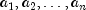. From this classification variable, RegressorsForGLM creates n indicator variables. For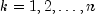, we have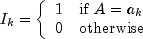For each classification variable, another set of variables is created from the indicator variables. These new variables are called dummy variables. Dummy variables are generated from the indicator variables in one of three manners:
1. The dummies are the n indicator variables.
2. The dummies are the first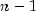indicator variables.
3. Thedummies are defined in terms of the indicator variables so that for balanced data, the usual summation restrictions are imposed on the regression coefficients.
In particular, for dummy method ALL, the dummy variables are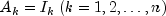. For dummy method LEAVE_OUT_LAST, the dummy variables are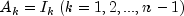. For dummy method SUM_TO_ZERO, the dummy variables are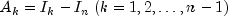. The regressors generated for an effect composed of a single-classification variable are the associated dummy variables.

Let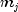be the number of dummies generated for the j-th classification variable. Suppose there are two classification variables A and B with dummies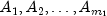and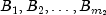The regressors generated for an effect composed of two classification variables A and B are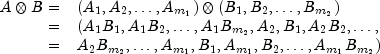More generally, the regressors generated for an effect composed of several classification variables and several continuous variables are given by the Kronecker products of variables, where the order of the variables is specified in setEffects. Consider a data matrix containing classification variables in Columns 0 and 1 and continuous variables in Columns 2 and 3. Label these four columns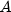,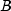,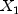, and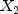. The regressors generated by the effect indices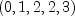are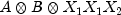.

Remarks

Let the data matrix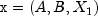, where A and B are classification variables andis a continuous variable. The model containing the effects, B, AB,,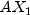,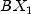, and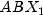is specified by setting nClassVariables=2 in the constructor and calling setEffects(effects), with

int effects[][] = { {0}, {1}, {0, 1}, {2}, {0, 2}, {1, 2}, {0, 1, 2} };

For this model, suppose that variable A has two levels,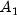and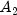, and that variable B has three levels,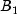,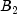, and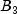. For each dummy method option, the regressors in their order of appearance in regressors are given below.

 dummyMethod Regressors ALL,,,,,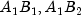,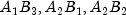,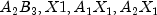,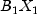,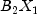,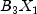,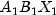,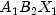,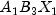,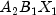,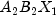,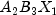LEAVE_OUT_LAST,,,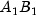,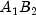,,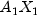,,,,SUM_TO_ZERO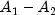,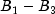,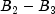,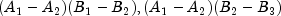,,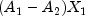,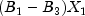,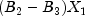,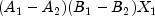,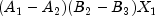Within a group of regressors corresponding to an interaction effect, the indicator variables composing the regressors vary most rapidly for the last classification variable, next most rapidly for the next to last classification variable, etc.

By default, RegressorsForGLM internally generates values for effects which correspond to a first order model with nEffects = nContinuousVariables + nClassVariables, where nContinuousVariables is the number of continuous variables and nClassVariables is the number of classification variables. The variables then are used to create the regressor variables. The effects are ordered such that the first effect corresponds to the first column of x, the second effect corresponds to the second column of x, etc. A second order model corresponding to the columns (variables) of x is generated if setModelOrder(2) is used.

The effects array for a first or second order model can be obtained by first using setModelOrder followed by getEffects. This array can then be modified and used as the argument to setEffects. This may be an easier way of setting the effects for an almost linear or quadratic model than creating the effects array from scratch.

There are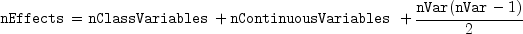effects, where nVar = nClassVariables+nContinuousVariables. The first nVar effects correspond to the columns of x, such that the first effect corresponds to the first column of x, the second effect corresponds to the second column of x, ..., the nVar-th effect corresponds to the nVar-th column of x (i.e. x[nVar-1]). The next nContinuousVariables effects correspond to squares of the continuous variables. The last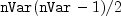effects correspond to the two-variable interactions.

• Let the data matrix, where A and B are classification variables andis a continuous variable. The effects generated and order of appearance is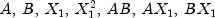• Let the data matrix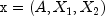, where A is a classification variable andandare continuous variables. The effects generated and order of appearance is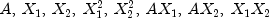• Let the data matrix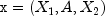, where A is a classification variable andandare continuous variables. The effects generated and order of appearance is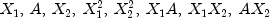Higher-order and more complicated models can be specified using setEffects.
Example 1, Example 2, Serialized Form
• Field Summary

Fields
Modifier and Type Field and Description
static int ALL
The n indicator variables are the dummy variables.
static int LEAVE_OUT_LAST
The dummies are the first n-1 indicator variables.
static int SUM_TO_ZERO
Thedummies are defined in terms of the indicator variables so that for balanced data, the usual summation restrictions are imposed on the regression coefficients.
• Constructor Summary

Constructors
Constructor and Description
RegressorsForGLM(double[][] x, int nClassVariables)
Constructor where the class columns are the first columns.
RegressorsForGLM(double[][] x, int[] classColumns)
Constructor with an explicit set of class column indicies.
• Field Detail

• ALL

public static final int ALL
The n indicator variables are the dummy variables.
Constant Field Values
• LEAVE_OUT_LAST

public static final int LEAVE_OUT_LAST
The dummies are the first n-1 indicator variables.
Constant Field Values
• SUM_TO_ZERO

public static final int SUM_TO_ZERO
Thedummies are defined in terms of the indicator variables so that for balanced data, the usual summation restrictions are imposed on the regression coefficients.
Constant Field Values
• Constructor Detail

• RegressorsForGLM

public RegressorsForGLM(double[][] x,
int nClassVariables)
Constructor where the class columns are the first columns.
Parameters:
x - is an nObservations by nClassVariables+nContinuousVariables array containing the data, where nObservations is the number of observations. The columns must be ordered such that the first nClassVariables columns contain the class variables and the next nContinuousVariables columns contain the continuous variables.
nClassVariables - is number of class variables. The number of continuous variables is assumed to be the number of columns in x-nClassVariables.
• RegressorsForGLM

public RegressorsForGLM(double[][] x,
int[] classColumns)
Constructor with an explicit set of class column indicies.
Parameters:
x - is an nObservations by nClassVariables+nContinuousVariables array containing the data. The columns containing the class variables are specified by classColumns.
classColumns - is an array containing the columns indices, in x, of the class variables.
• Method Detail

• getDummyMethod

public int getDummyMethod()
Returns the dummy method.
Returns:
One of ALL (the default), LEAVE_OUT_LAST or SUM_TO_ZERO.
• getEffects

public int[][] getEffects()
Returns the effects.
Returns:
a jagged array containing the effects. The number of rows in the matrix is the number of effects. For each row, the values are the 0-based column numbers of x.
• getEffectsColumns

public int[][] getEffectsColumns()
Returns a mapping of effects to regressor columns.
Returns:
A jagged int array. The number of rows is equal to the number of effects. Each row contains the column numbers of the regressor matrix into which the corresponding effect is mapped.
• getNumberOfMissingRows

public int getNumberOfMissingRows()
Returns the number of rows in the regressors matrix containing NaN (not a number). A row of the regressors matrix contains NaN for a regressor when any of the variables involved in generation of the regressor equals NaN or if a value of one of the classification variables in the model is not given by effects.
Returns:
The number of rows in the data matrix having missing data.
• getNumberOfRegressors

public int getNumberOfRegressors()
Returns the number regressors.
Returns:
The number of regressors. This is the number of columns in the regressor matrix.
• getRegressors

public double[][] getRegressors()
Returns the regressor array.
Returns:
An array of size number of observations by number of regresssors.
• setDummyMethod

public void setDummyMethod(int dummyMethod)
Sets the dummy method.
Parameters:
dummyMethod - must be one of ALL (the default), LEAVE_OUT_LAST or SUM_TO_ZERO.
• setEffects

public void setEffects(int[][] effects)
Set the effects. This overrides any previously set model order.
Parameters:
effects - is a jagged array. The number of rows in the matrix is the number of effects. For each row, the values are the 0-based column numbers of x.
• setModelOrder

public void setModelOrder(int modelOrder)
Sets the order of the model. Model order can be specified as 1 or 2. Use setEffects to specify more complicated models. This overrides previously set effects.
Parameters:
modelOrder - is one or two. The default effects are equivalent to model equal to one.
JMSLTM Numerical Library 7.2.0